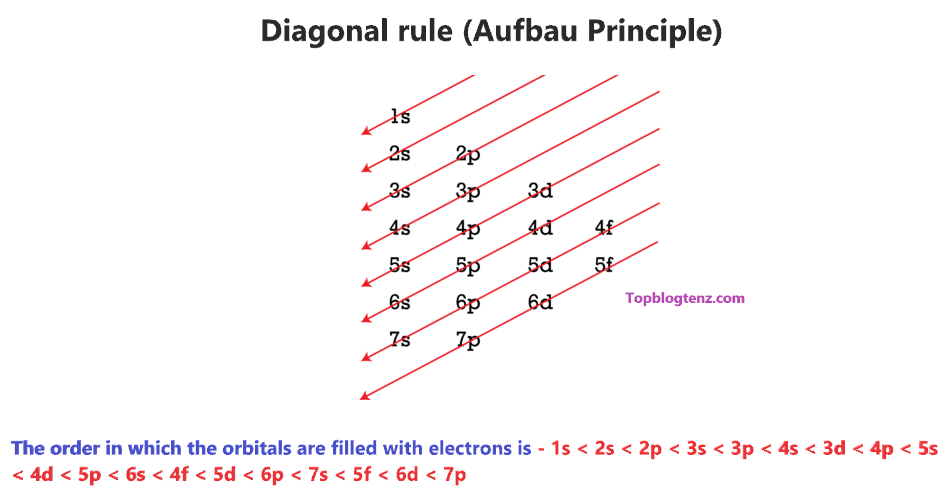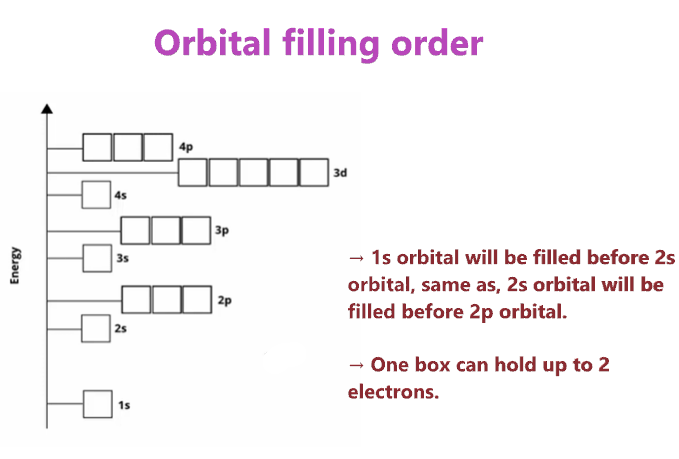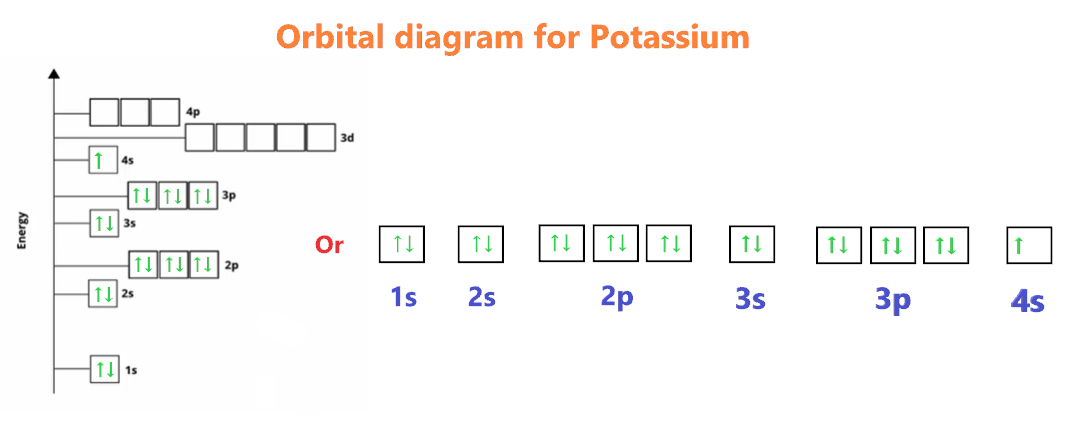# orbital filling diagram calculator

Home > Chemistry Calculators > orbital diagram calculator

An orbital diagram calculator is an online tool to get the orbital diagram of an atom. The orbital diagram is simply a pictorial representation of the arrangement of electrons in the orbital of an atom, it shows the electrons in the form of arrows, and also, indicates the spin of electrons.

The orbital diagram calculator contains the list of the first 20 elements, pick any of these by clicking on the dropdown box, and get an orbital diagram or notation for the selected atom.

## How to Calculate Orbital Diagrams?

The orbital diagram simply represents the arrangement of electrons in the different orbitals of an atom, it uses an arrow to represent the electrons, every orbital(one box) contains a maximum of 2 electrons.

There are three rules followed for calculating the orbital diagram for an atom.

(1). Aufbau’s principle:- This rule state that the lower energy orbital will be filled before the higher energy orbital, for example – the 1s orbital will fill before the 2s orbital.

(2). Hund’s rule:- This rule state that each orbital of a given subshell should be filled with one electron each before pairing them. That means “Each orbital gets one electron first, before adding the second electron to the orbital”.

(3). Pauli Exclusion Principle:- This rule state that, no two electrons can occupy the same orbital with the same spin. That means “One must be spin up (↑) and one must be spin down (↓)”.If you understand the above rules then calculating the orbital diagram or orbital notation for any atom is super easy.

Basics of Orbital diagram:-

There are different types of orbitals – s, p, d, and, f. These orbitals contain a number of boxes that can hold a number of electrons. Let’s see.

Each box will hold a maximum of 2 electrons with opposite spin.

• S orbital contains 1 box that can hold a maximum of 2 electrons.
• P orbital contains 3 boxes that can hold a maximum of 6 electrons.
• D orbital contains 5 boxes that can hold a maximum of 10 electrons.
• F orbital contains 7 boxes that can hold a maximum of 14 electrons.

The orbital diagram will be filled in the same order as described by the Aufbau principle.

“The Aufbau principle states that electrons will inhabit the lowest-energy orbitals first. This means that electrons can only enter higher-energy orbitals after lower-energy orbitals have been entirely filled.”We have a diagonal rule for electron filling order in the different subshells using the Aufbau principle.So, the order in which the orbitals are filled with electrons from lower energy to higher energy is –

1s < 2s < 2p < 3s < 3p < 4s < 3d < 4p < 5s < 4d < 5p < 6s < 4f < 5d < 6p < 7s < 5f < 6d < 7p and so on.

Trick:- you can learn the order of the orbital box diagram and then fill out the electrons in it.## How to calculate an Orbital diagram/notation for any atom?

To calculate the orbital diagram for an atom, follow these basic steps-

1. Find the number of electrons in an atom.
2. Write the electron configuration for an atom to determine which orbitals should be filled.
3. Fill the electrons in empty boxes using three principles – Aufbau, Hund’s, and Pauli Exclusion.

Let’s take an example of the Nitrogen atom to understand the concept of the making of filling the orbital diagram.

### How to calculate an Orbital diagram for Nitrogen?

We know that the nitrogen atom has a total of 7 electrons that need to be placed into orbitals, now for calculating its orbital diagram, we need to show its electrons in form of an arrow in different boxes using Aufbau, Hund’s, and Pauli’s exclusion rule.

• Nitrogen has a total of 7 electrons and its electron configuration is 1s22s22p3.
• As per the Aufbau rule, the electrons will be filled into 1s orbital first then 2s, then 2p…so on.
• 1s orbital contains 1 box, 2s orbital also contains 1 box and 2p orbital contains 3 boxes. Also, one box can hold up to two electrons.
• Therefore, the first two electrons of nitrogen will go in the 1s orbital, and the next two will go in the 2s orbital, now we are left with 3 electrons.
• These 3 electrons will go in the 2p orbital, since, the 2p orbital has 3 boxes, so, these electrons will be filled using Hund’s rule. (Each box gets one electron first).Let’s take one more example to understand the procedure of calculating the orbital diagram for an atom.

### How to calculate an Orbital diagram for Potassium?

We know the potassium has a total of 19 electrons that need to be placed into orbitals, now for calculating its orbital diagram, we need to show its electrons in form of an arrow in different boxes using Aufbau, Hund’s, and Pauli’s exclusion rule.

• Potassium has a total of 19 electrons and its electron configuration is 1s22s22p63s23p64s1.
• As per the Aufbau rule, the electrons will be filled into 1s orbital first then 2s, then 2p…so on.
• 1s orbital contains 1 box, 2s orbital also contains 1 box, 2p orbital contains 3 boxes, 3s orbital contains 1 box, 3p orbital contains 3 boxes, and 4s orbital contains 1 box.  Also, one box can hold up to two electrons.
• Therefore, the first two electrons of potassium will go into the 1s orbital, the next two will go into the 2s orbital, and after that, the next six electrons will go into the 2p orbital, since, the 2p orbital has 3 boxes.
• After that, the next two electrons will go in the 3s orbital, and the next six electrons will enter the 3p orbital. Now, the 3p orbital is full.
• Therefore, the remaining one electron will go in 4s orbital in the clockwise direction. (↑)Name of the Elements Orbitals Hydrogen orbital diagram Consists of one orbital – 1s. Helium orbital diagram Consists of one orbital – 1s. Lithium orbital diagram Consists of two orbitals – 1s and 2s. Beryllium orbital diagram Consists of two orbitals – 1s and 2s. Boron orbital diagram Consists of three orbitals – 1s, 2s, and, 2p Carbon orbital diagram Consists of three orbitals – 1s, 2s, and, 2p Nitrogen orbital diagram Consists of three orbitals – 1s, 2s, and, 2p Oxygen orbital diagram Consists of three orbitals – 1s, 2s, and, 2p Fluorine orbital diagram Consist of three orbitals – 1s, 2s, and, 2p Neon orbital diagram Consists of three orbitals – 1s, 2s, and, 2p Sodium orbital diagram Consists of four orbitals – 1s, 2s, 2p, and, 3s. Magnesium orbital diagram Consists of four orbitals – 1s, 2s, 2p, and, 3s. Aluminum orbital diagram Consists of five orbitals – 1s, 2s, 2p, 3s, and 3p. Silicon orbital diagram Consists of five orbitals – 1s, 2s, 2p, 3s, and 3p. Phosphorus orbital diagram Consists of five orbitals – 1s, 2s, 2p, 3s, and 3p. Sulfur orbital diagram Consists of five orbitals – 1s, 2s, 2p, 3s, and 3p. Chlorine orbital diagram Consists of five orbitals – 1s, 2s, 2p, 3s, and 3p. Argon orbital diagram Consists of five orbitals – 1s, 2s, 2p, 3s, and 3p. Potassium orbital diagram Consists of six orbitals – 1s, 2s, 2p, 3s, 3p, and 4s. Calcium orbital diagram Consists of six orbitals – 1s, 2s, 2p, 3s, 3p, and 4s.
 Check related Calculator Valence electron calculator Lewis structure calculator Electron configuration calculator Formal charge calculator

## FAQ

### What is an orbital diagram calculator?

An orbital diagram calculator is an online tool to determine the orbital diagram for an atom. This orbital diagram calculator contains the list of the first 20 elements, you can choose any of the atoms by clicking on the dropdown box, and you will get an orbital box diagram for a particular atom.### How do you calculate an Orbital diagram?

The orbital diagrams are calculate by following three basic rules – the Aufbau Principle rule, the Pauli-Exclusion Principle, and Hund’s rule. There are different types of orbitals – s, p, d, and, f. These orbitals contain a number of boxes that can hold a number of electrons.

### What is the order of filling electrons in the Orbital boxes diagram?

The orbital diagram will be filled in the same order as described by the Aufbau principle.

The order in which the orbitals are filled with electrons from lower energy to higher energy is –

1s < 2s < 2p < 3s < 3p < 4s < 3d < 4p < 5s < 4d < 5p < 6s < 4f < 5d < 6p < 7s < 5f < 6d < 7p and so on.

The above order means –

• 1s orbital will be filled first than 2s.
• Same as 2s orbital will be filled first than 2p orbital…..and so on.

Did you like it?

#### Vishal Goyal

Vishal Goyal is the founder of Topblogtenz, a comprehensive resource for students seeking guidance and support in their chemistry studies. He holds a degree in B.Tech (Chemical Engineering) and has four years of experience as a chemistry tutor. The team at Topblogtenz includes experts like experienced researchers, professors, and educators, with the goal of making complex subjects like chemistry accessible and understandable for all. A passion for sharing knowledge and a love for chemistry and science drives the team behind the website. Let's connect through LinkedIn: https://www.linkedin.com/in/vishal-goyal-2926a122b/

Share it...

Topblogtenz is a website dedicated to providing informative and engaging content related to the field of chemistry and science. We aim to make complex subjects, like chemistry, approachable and enjoyable for everyone.